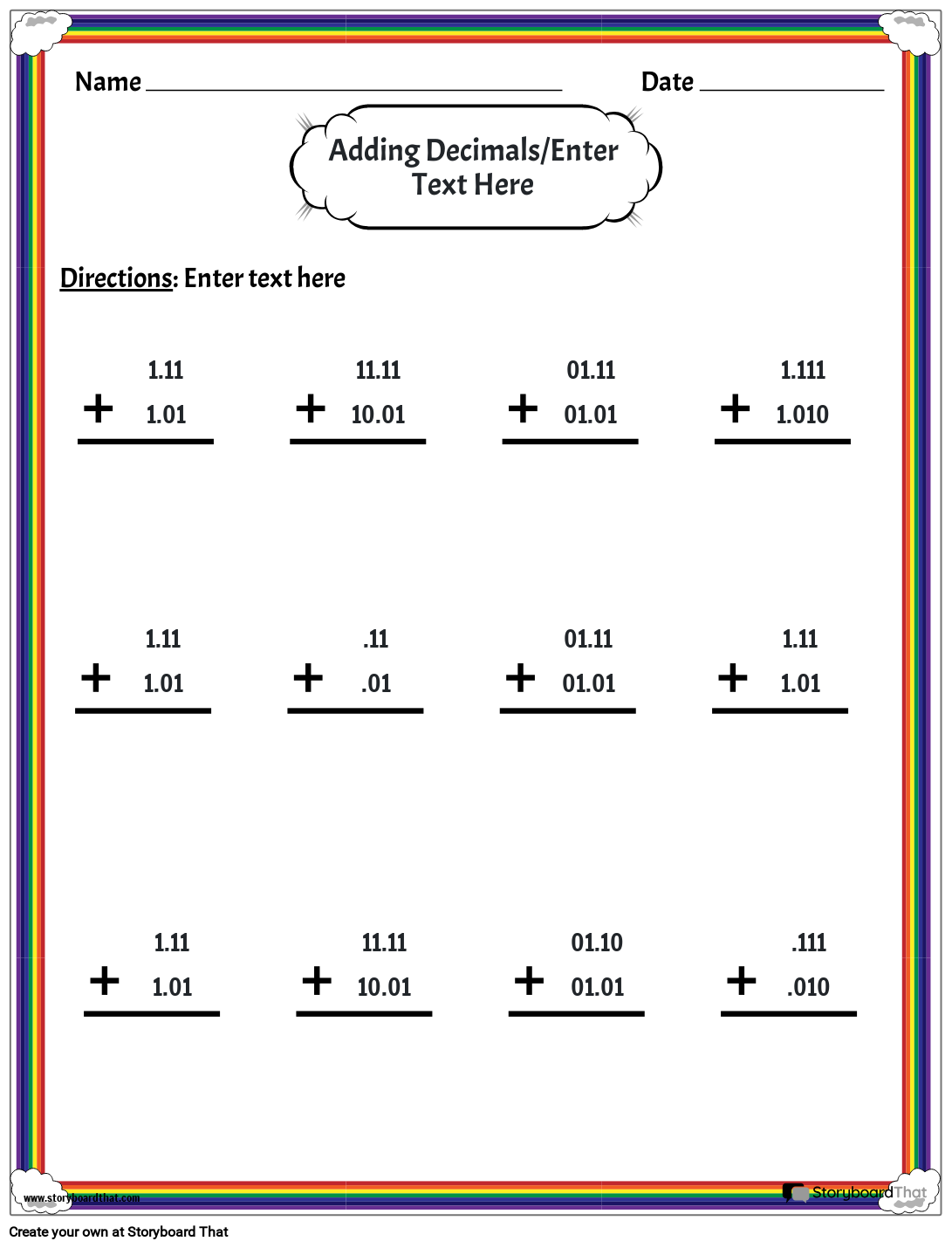Copy Template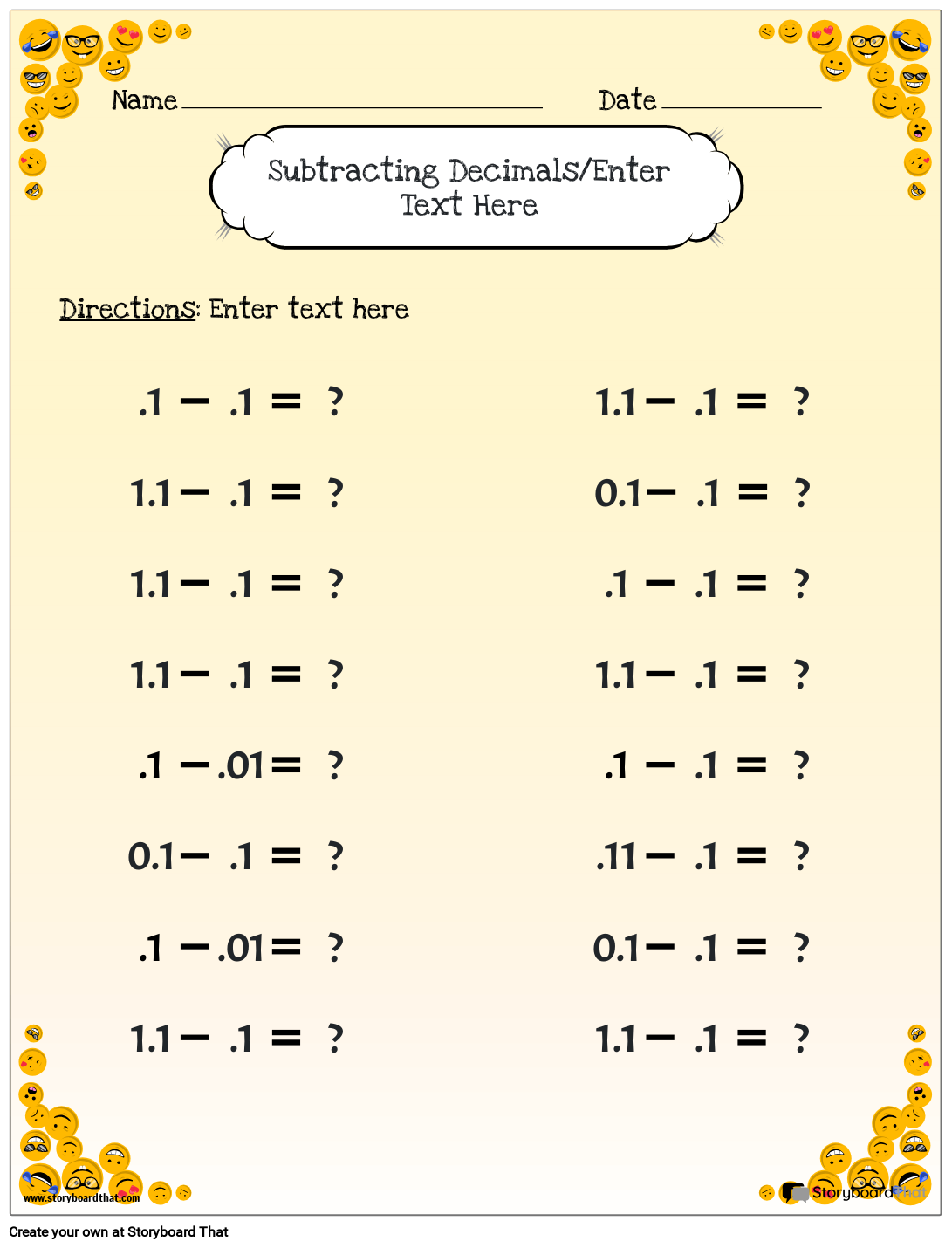Copy Template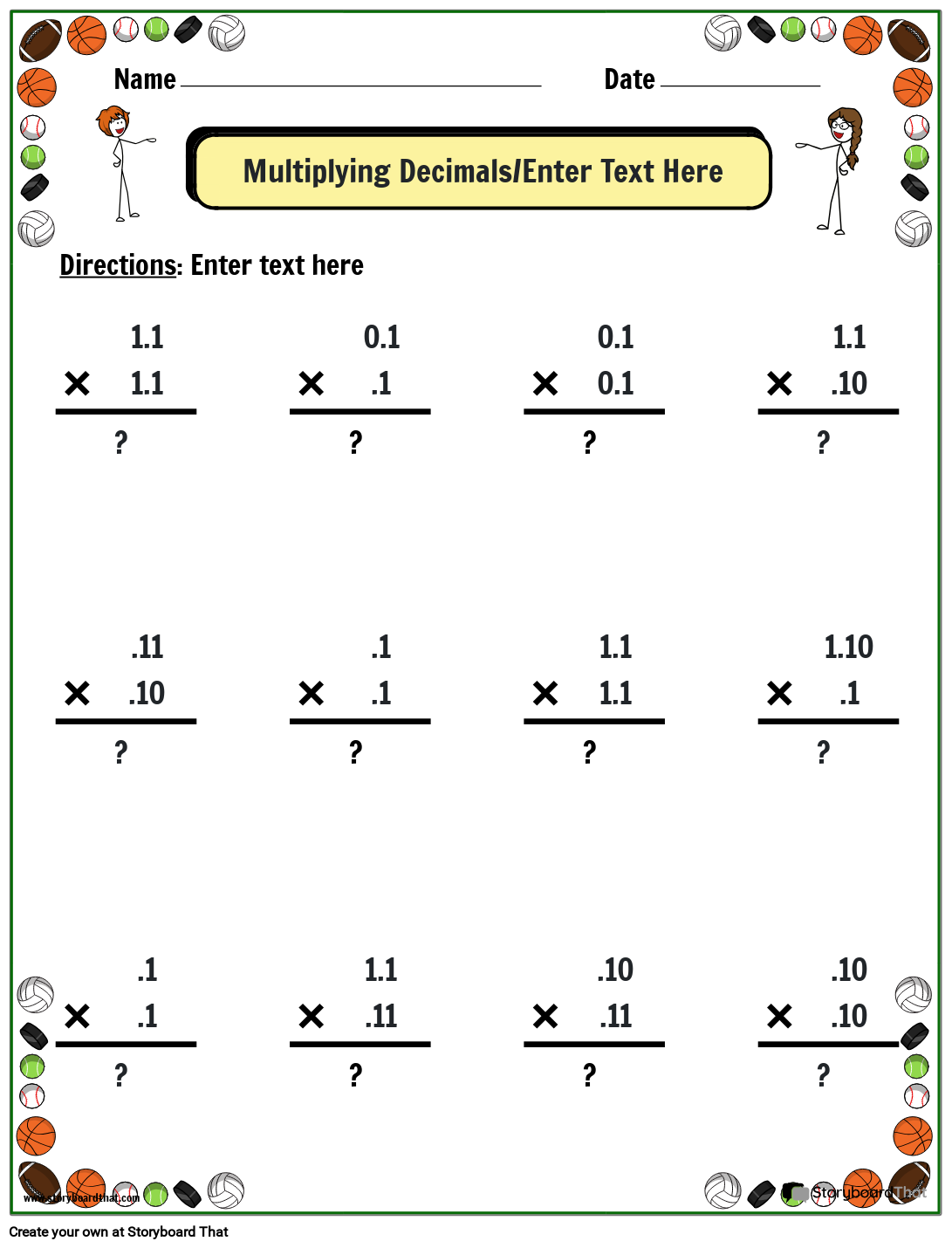Copy Template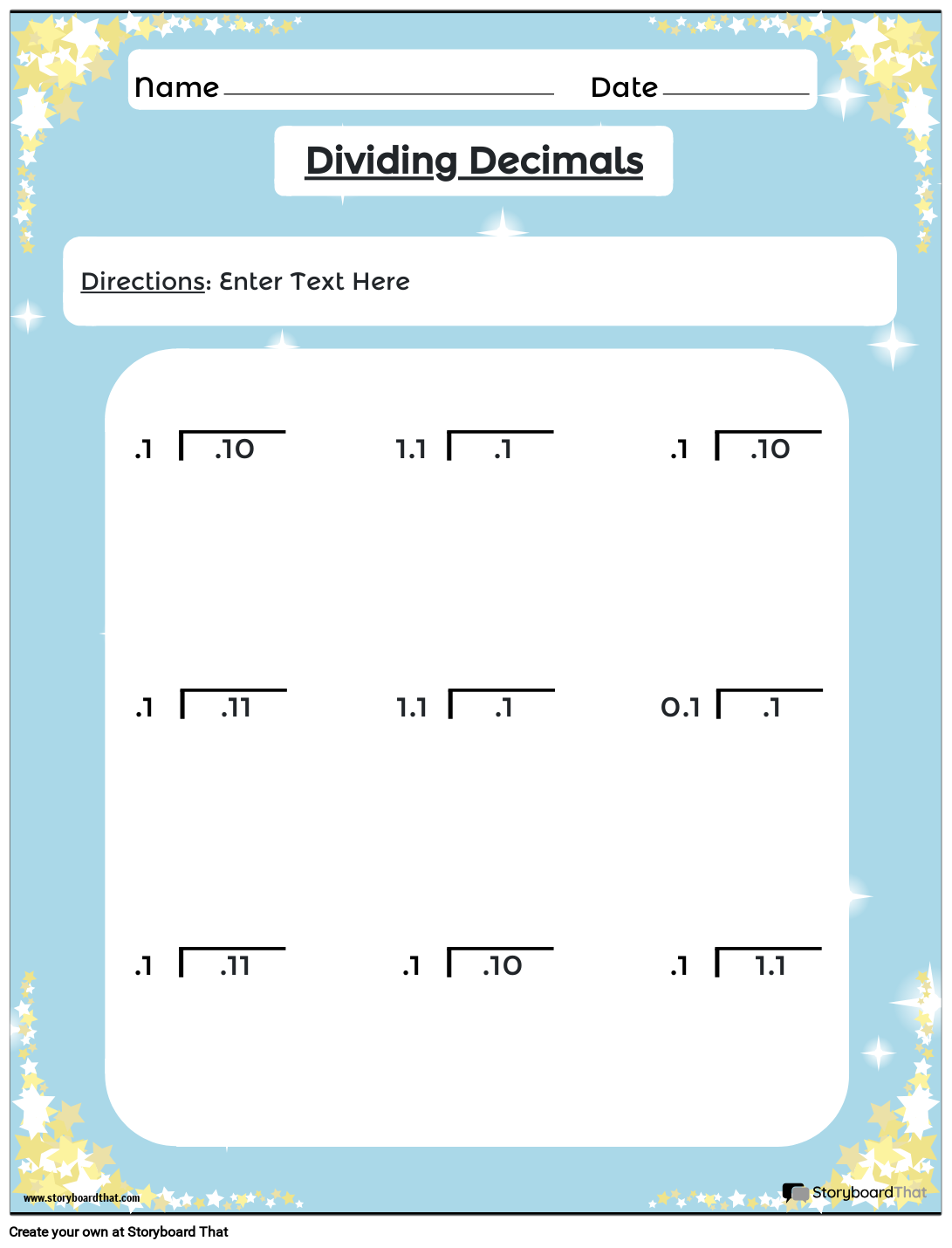Copy Template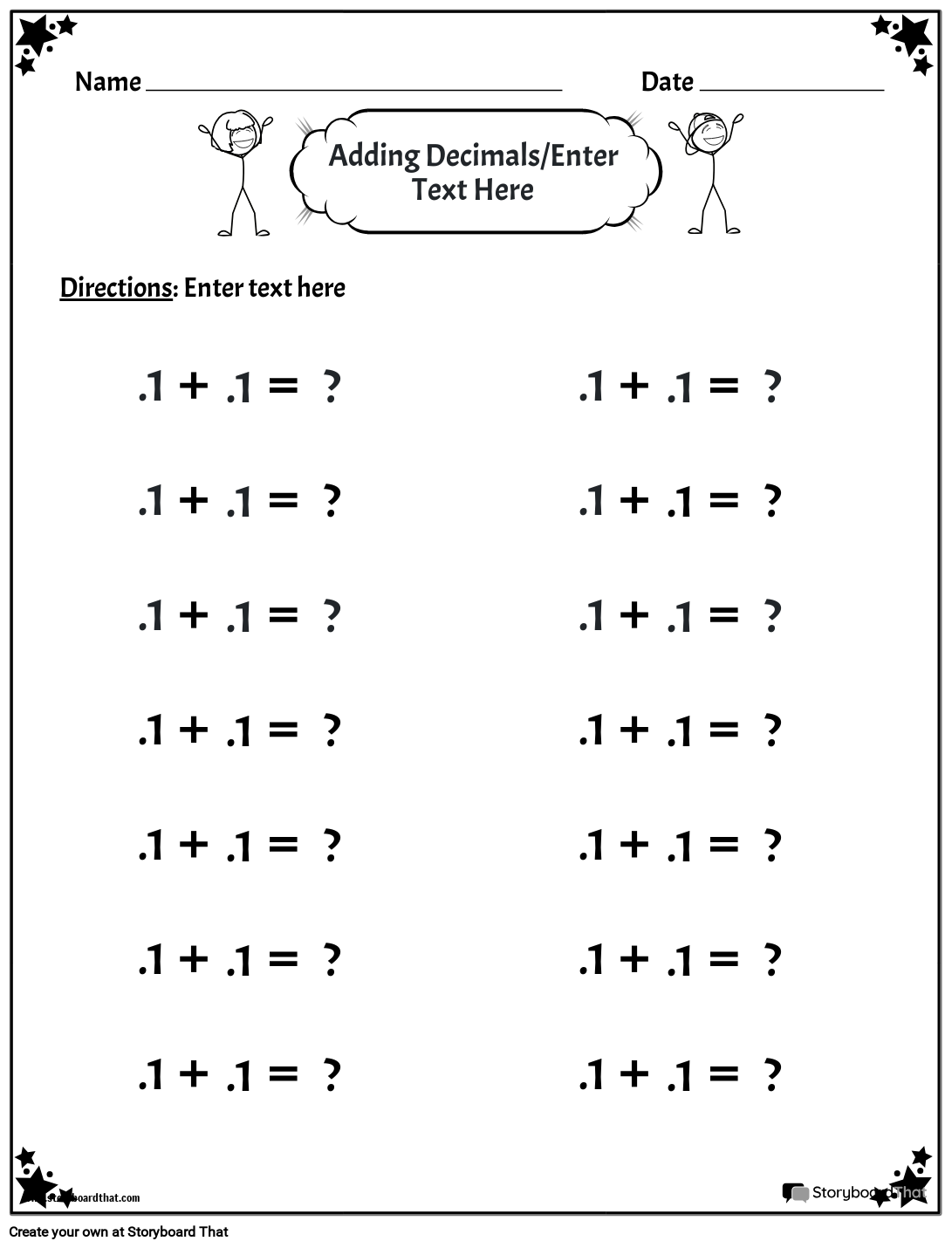Copy Template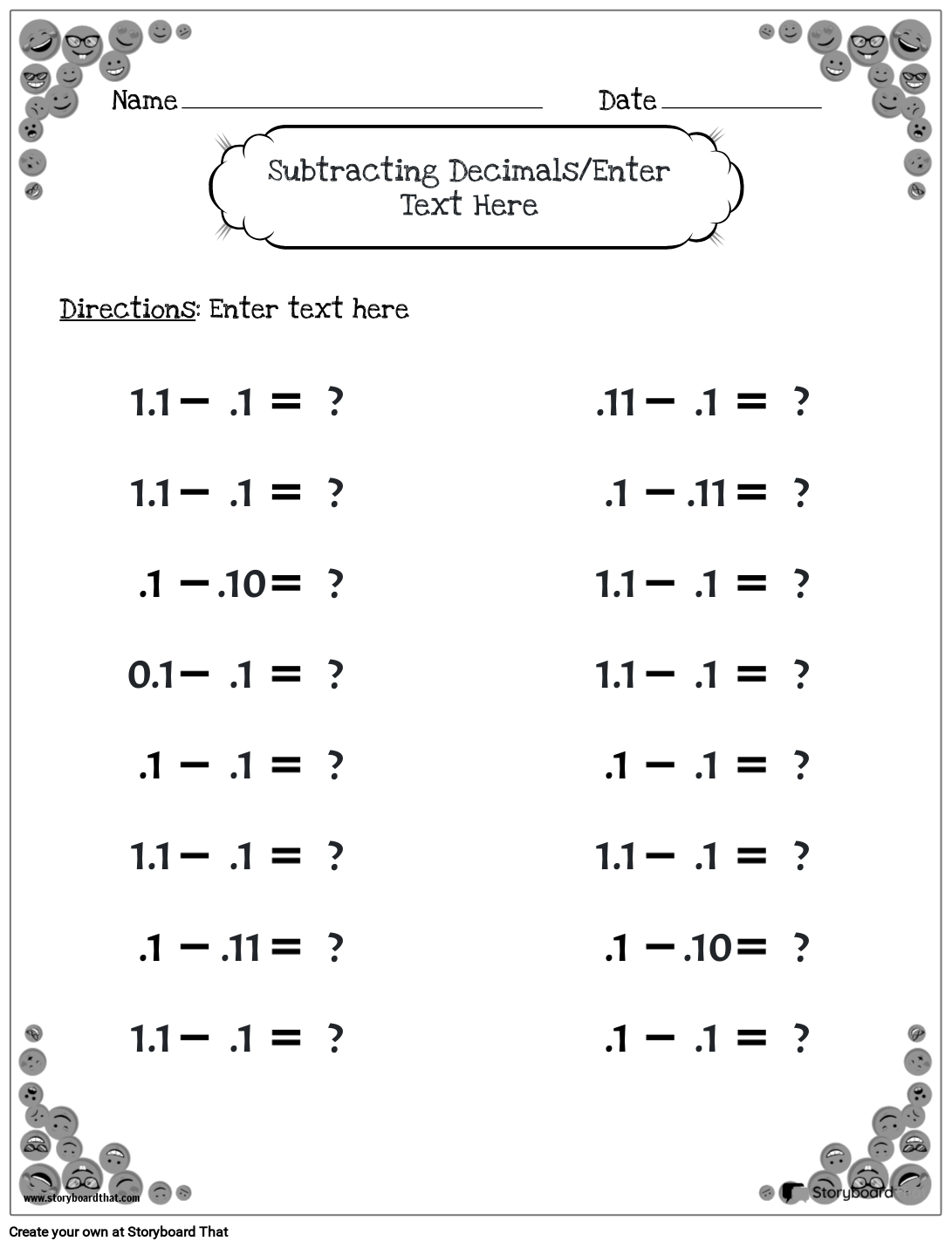Copy Template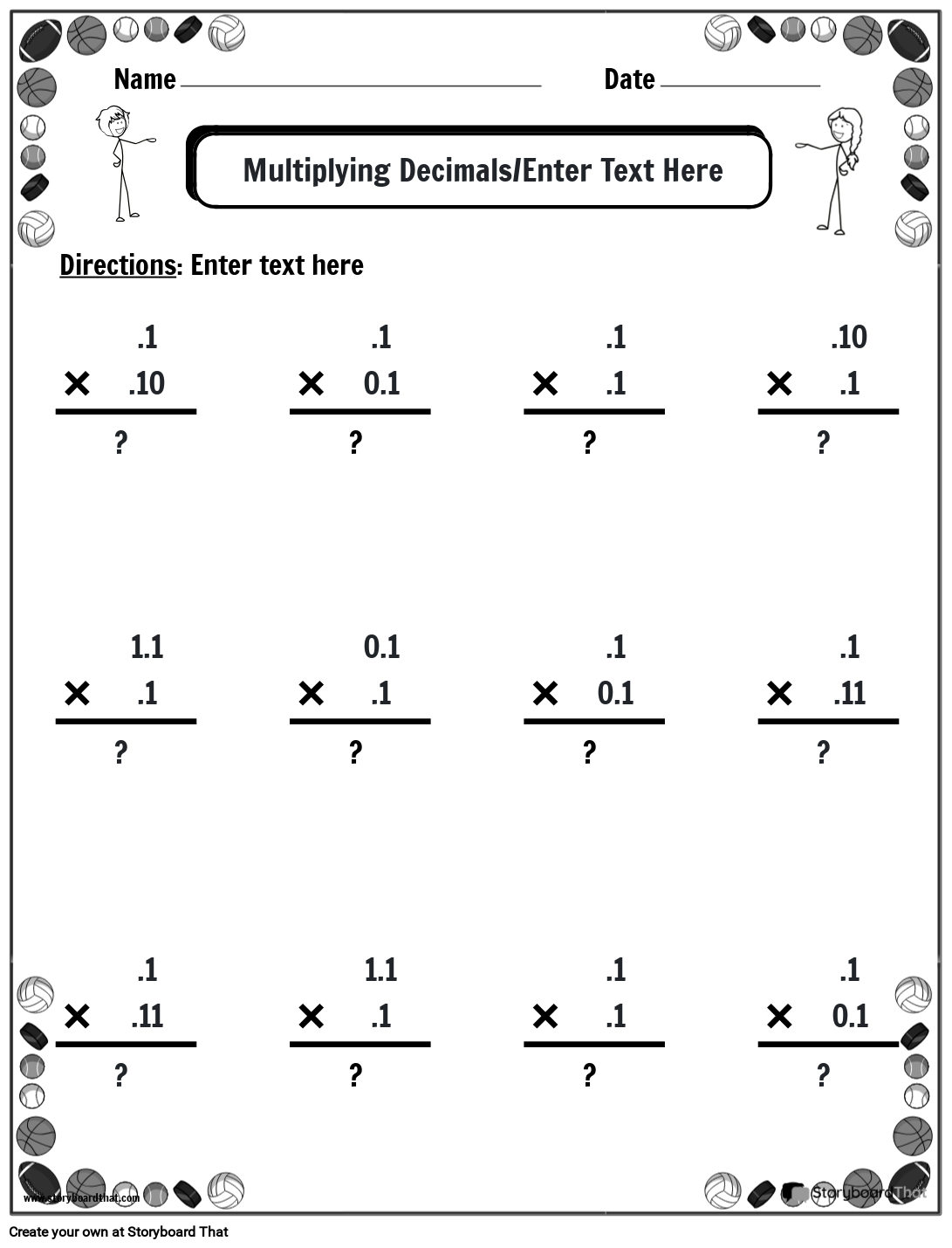Copy Template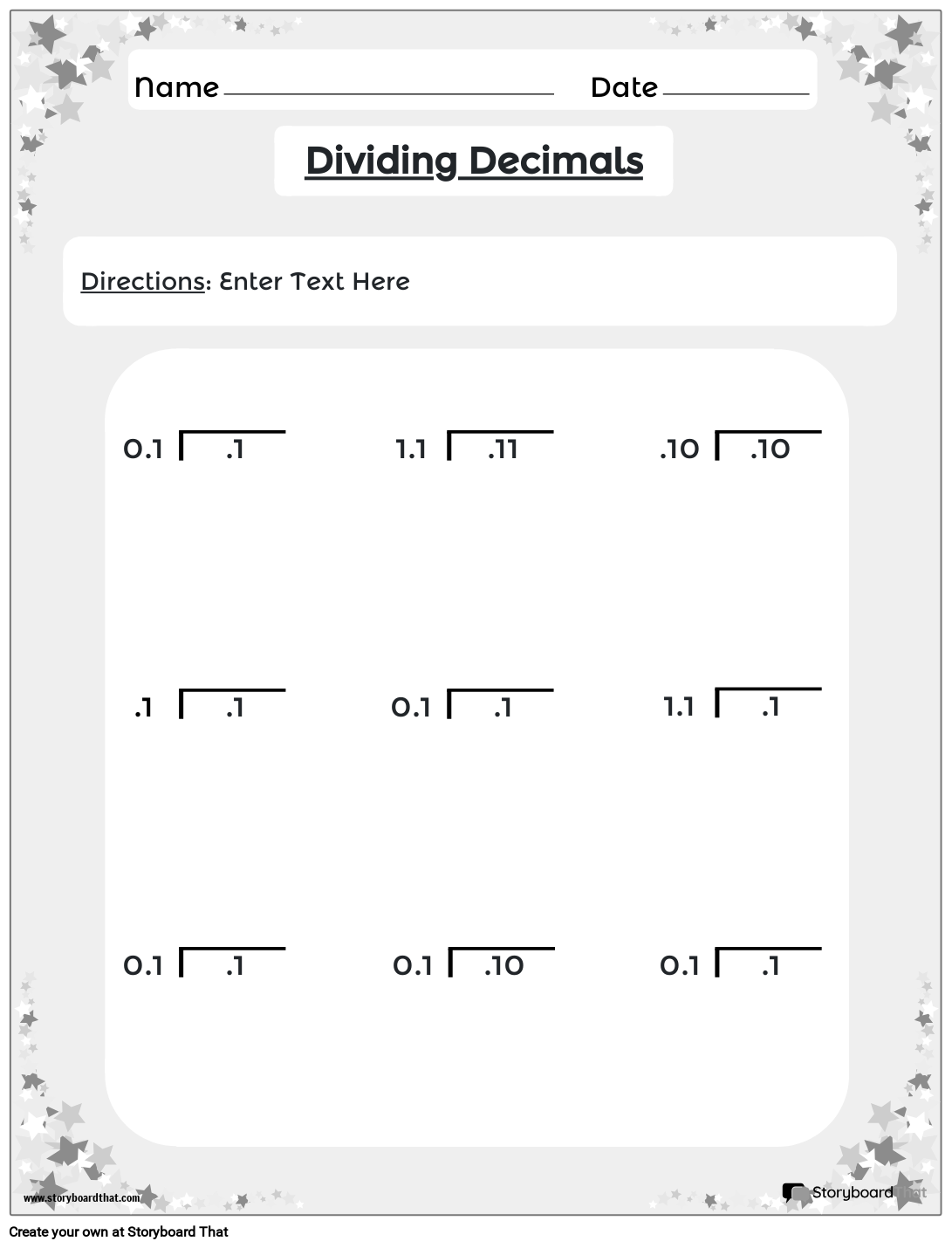Copy Template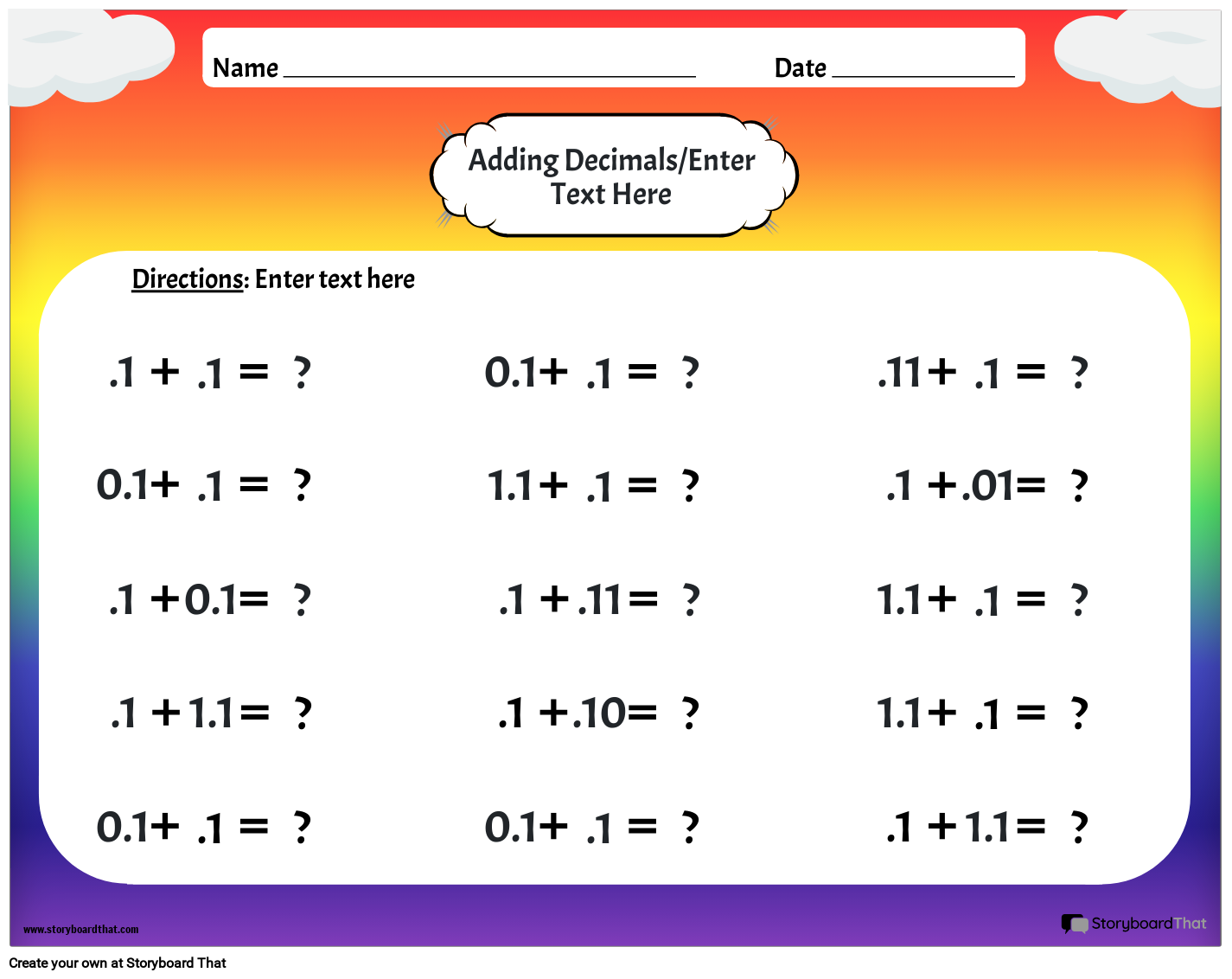Copy Template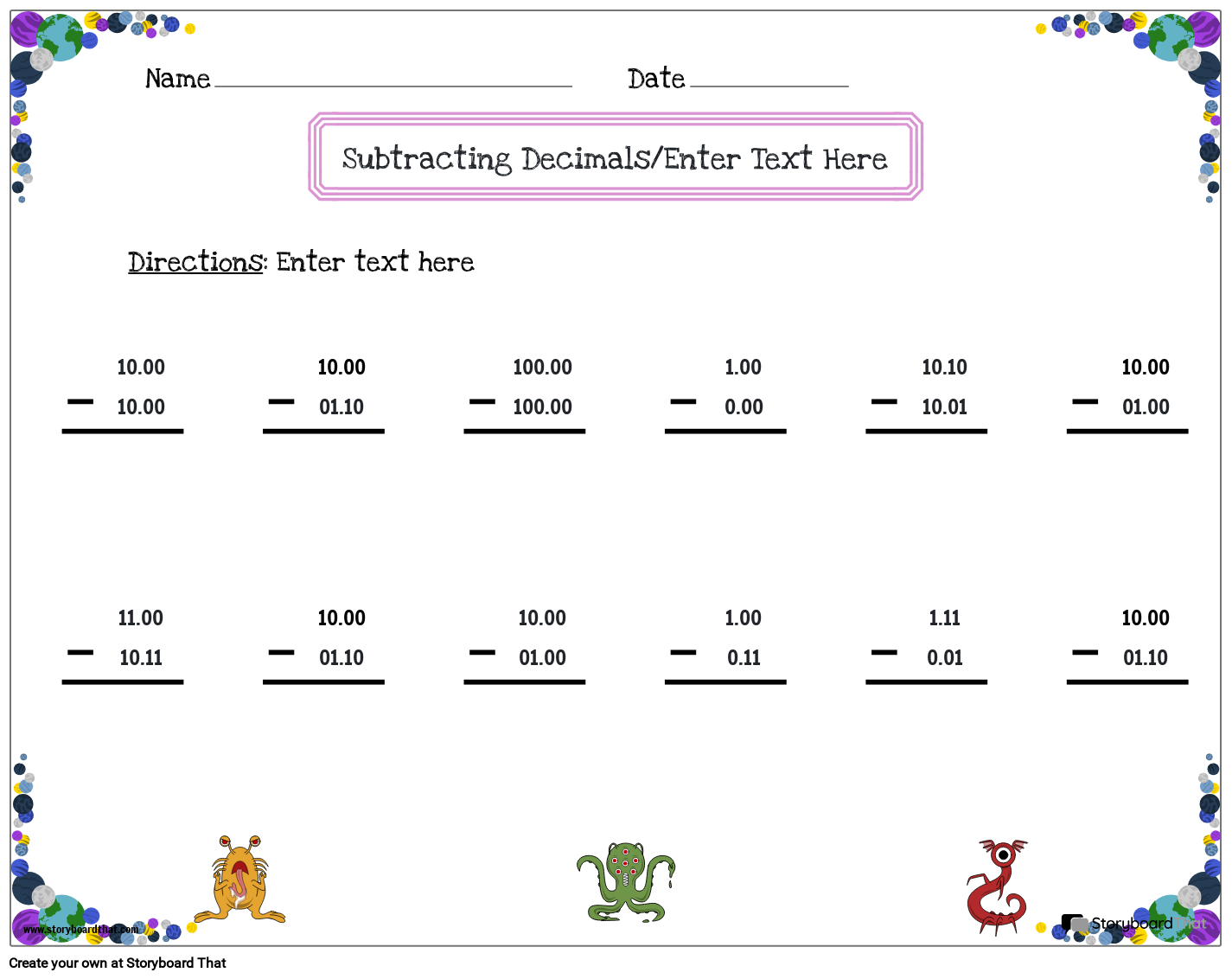Copy Template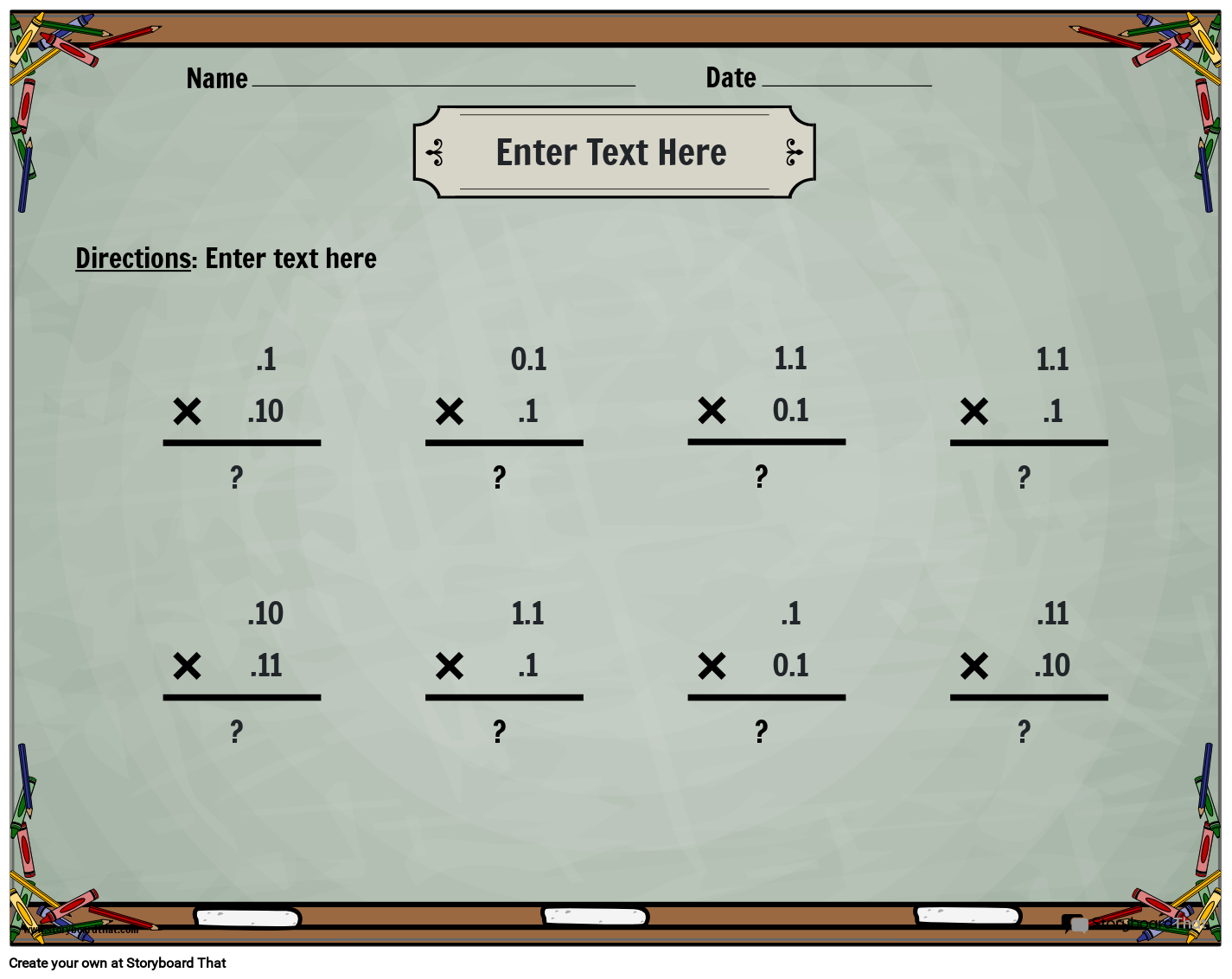Copy Template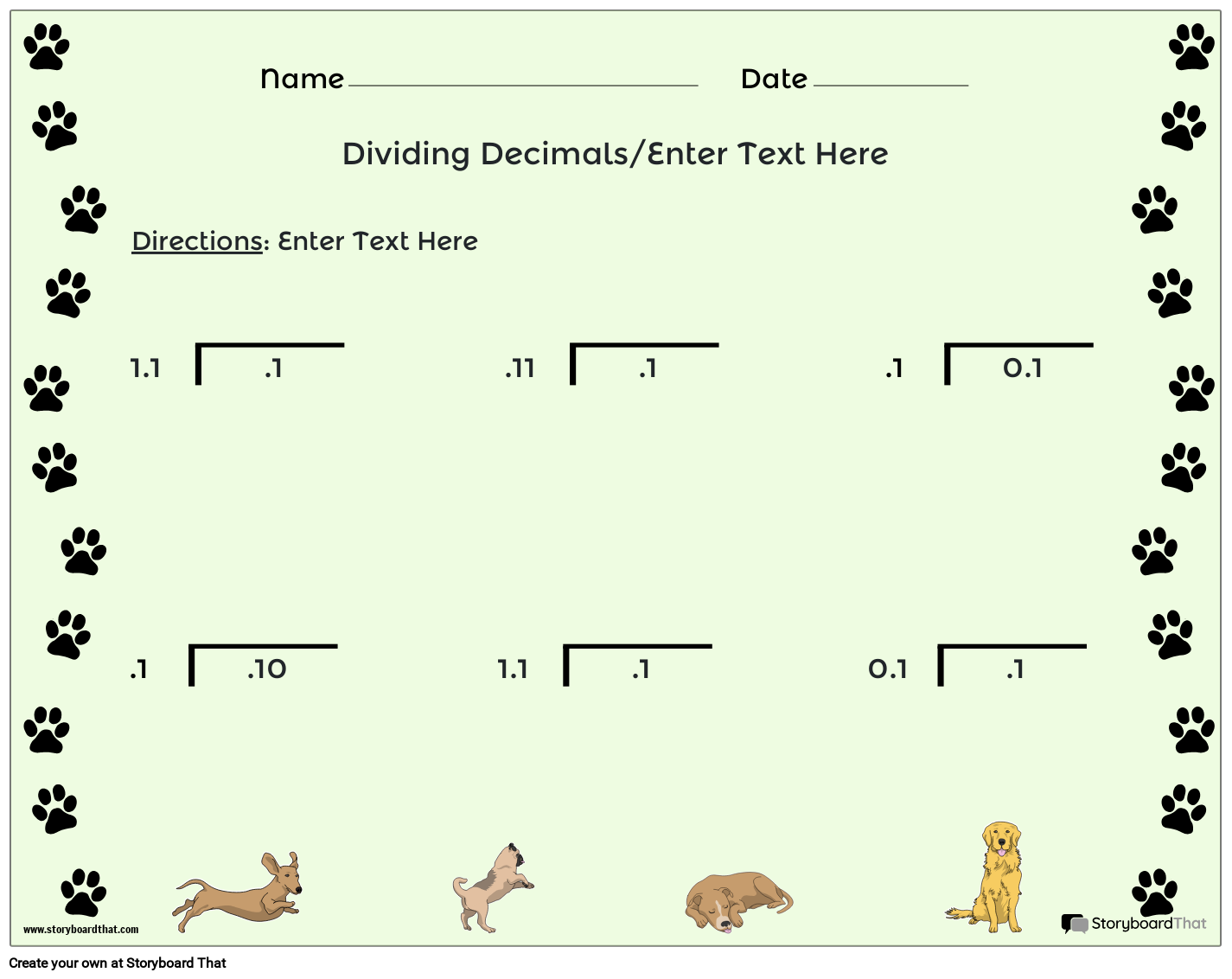Copy Template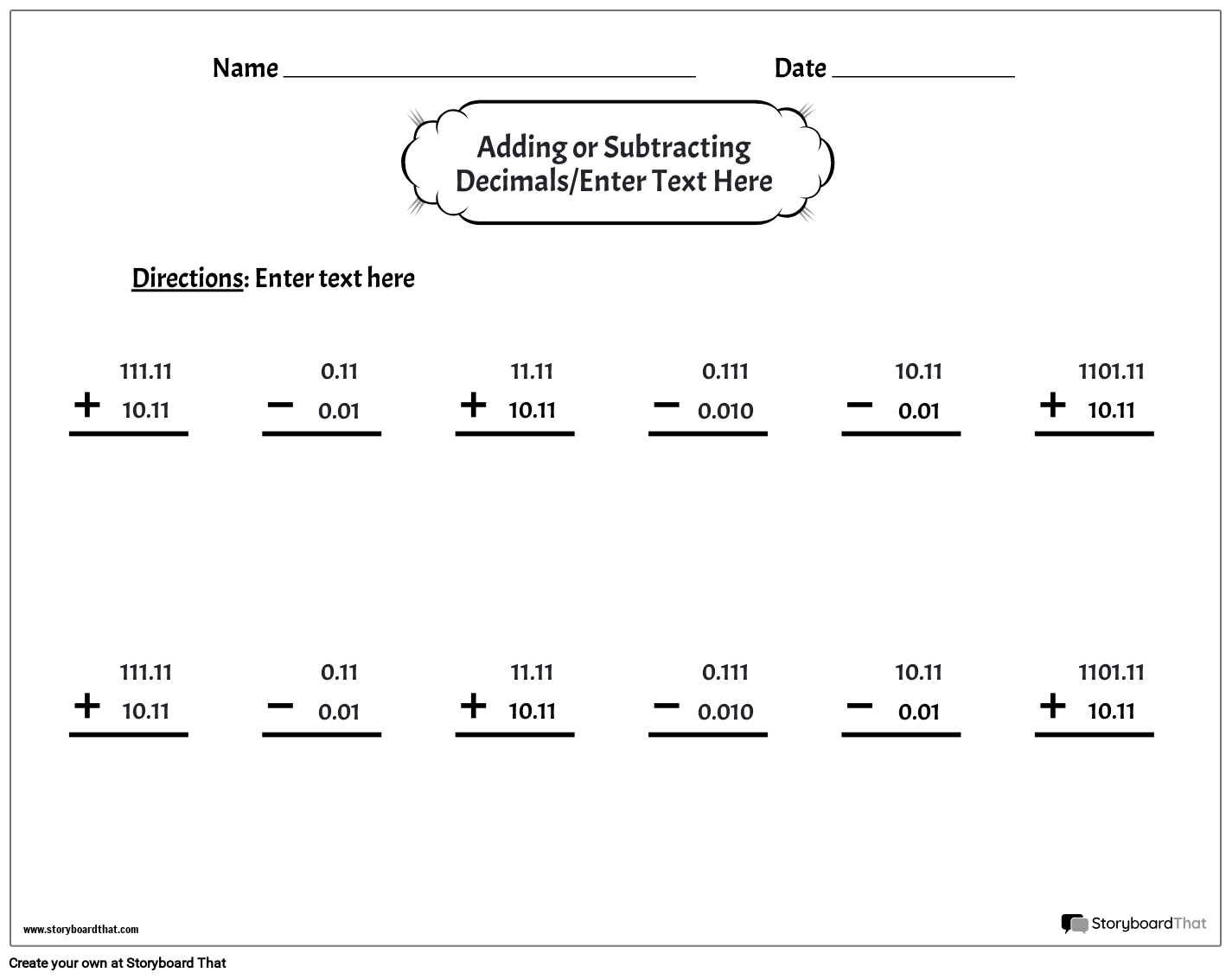Copy Template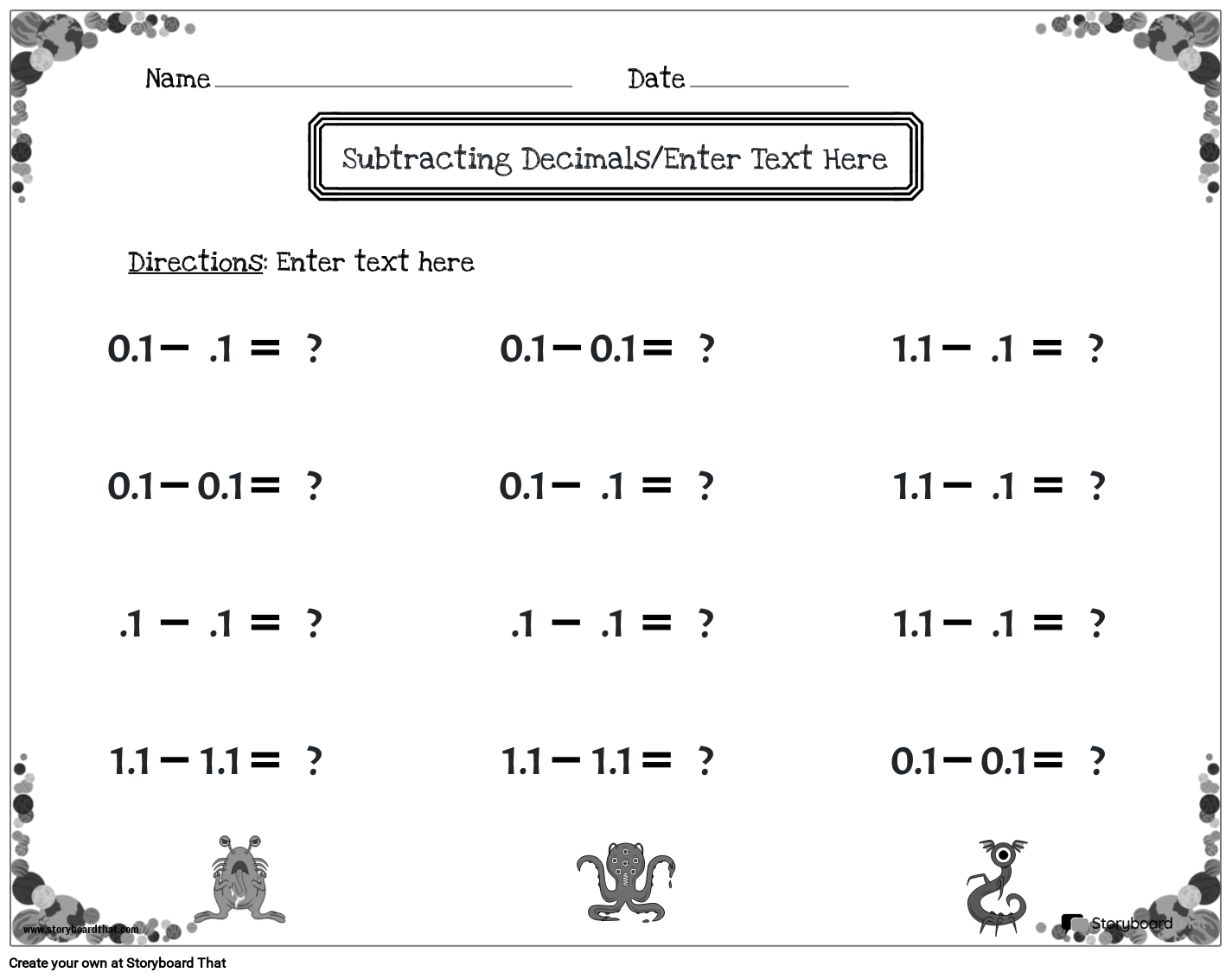Copy Template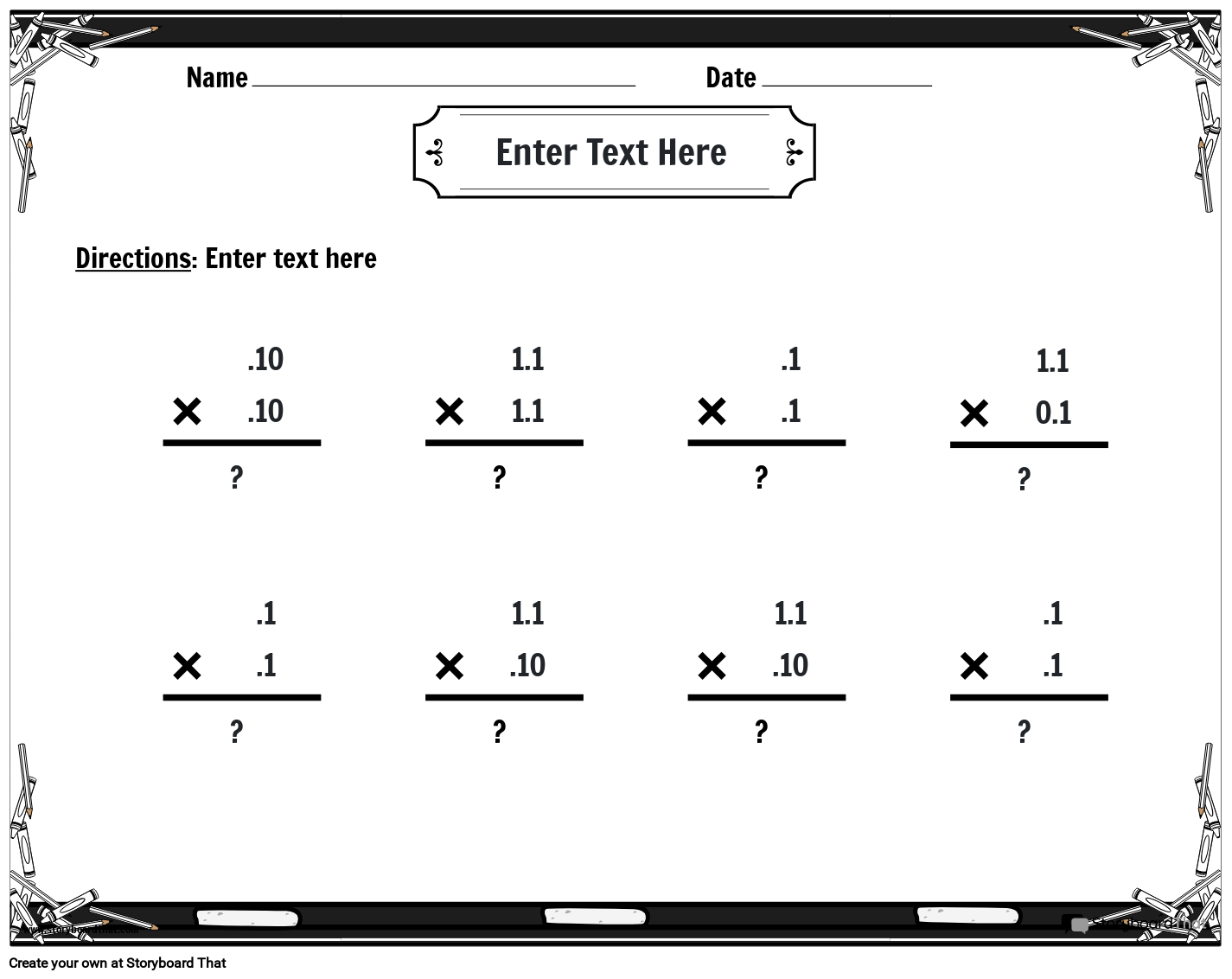Copy Template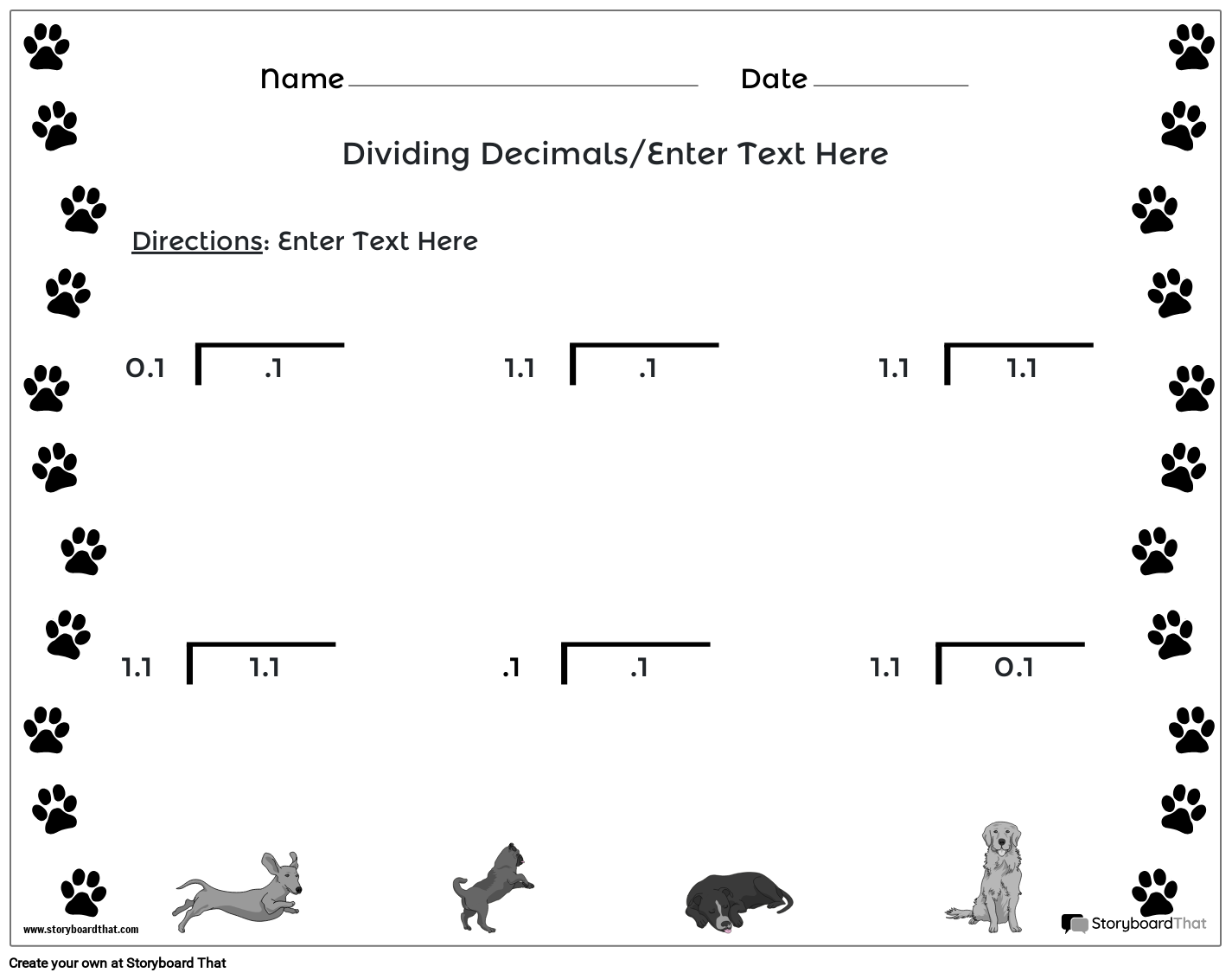Copy Template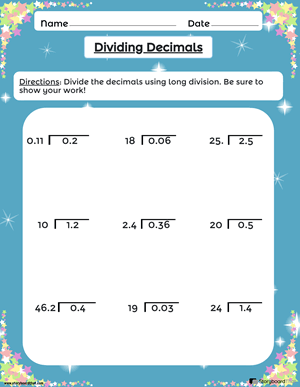## What is a Decimal?

A decimal number is a number that includes a decimal point, which separates the whole number part from the fractional part. It is a way to represent numbers that are not whole or integers. The decimal point is usually denoted by a period (.), and it indicates the division between the whole and fractional parts of a number.

In a decimal number, the digits to the left of the decimal point represent the whole number part, while the digits to the right of the decimal point represent the fractional part. Each digit to the right of the decimal point has a place value that is a power of 10, such as tenths place, hundredths place, thousandths place, and so on.

Decimal numbers are used in various real-life situations, such as measuring lengths, weights, or quantities that are not whole numbers. They allow for more precise and accurate calculations and provide a more detailed representation of quantities.

## Unlock the World of Decimals with Engaging Decimal Worksheets

Welcome to our comprehensive collection of decimal worksheets designed to help students unlock the world of decimals and develop their mathematical skills. Whether you are teaching adding and subtracting decimals, multiplying decimals, or dividing decimal numbers, our engaging decimal worksheets provide ample practice opportunities to reinforce these concepts.

With the use of our decimal practice worksheets, students can practice various operations, such as adding decimals, decimal subtraction, and decimal multiplication, and dividing decimals that will develop their proficiency in working with decimal numbers.

In addition, they will have the opportunity to solve word problems involving decimals and practice dividing decimals to solidify their knowledge. Our worksheets are designed to provide students with a solid foundation in decimal arithmetic and equip them with the necessary skills to tackle more complex decimal word problems.

We offer free decimal worksheets that can be easily accessed and downloaded, making them a valuable resource for both educators and students. Whether you need additional practice worksheets, homework assignments, or assessment tools, our collection has you covered. Each worksheet includes clear instructions and answer keys to facilitate independent learning and provide immediate feedback.

So, dive into our collection of math worksheets and discover the power of practice and reinforcement in mastering decimal operations. Whether your students are just starting to explore decimals or need extra practice to solidify their understanding, our worksheets offer a comprehensive and effective learning resource.

## How to Effectively Teach Decimals Using our Decimal Worksheets

Decimals are an essential part of the mathematics curriculum, and teaching them effectively is crucial for students' understanding and success in the subject. Our decimal worksheets provide a comprehensive and engaging resource to support teachers in their instruction. Here are some strategies to effectively teach decimals:

• Begin with Decimal Place Value Understanding: Before introducing operations, ensure that students have a solid understanding of place value and how the position of digits affects the value of a decimal. Reinforce the concept that the decimal point separates the whole number part from the decimal part.

• Introduce Decimal Adding and Subtracting: Start by using our decimal addition worksheets to help students understand the process of adding decimal numbers. Emphasize the importance of aligning the decimal points and carrying over when necessary. Similarly, our decimal subtraction worksheets can be used to teach the concept of subtracting decimals, including the regrouping process.

• Introduce Multiplying Decimals: Use our decimal multiplication worksheets to introduce students to the concept of multiplying decimals. Provide them with practice problems that involve multiplying numbers with varying decimal places. Guide students in correctly placing the decimal point in the product by counting the total number of decimal places in the factors.

• Explore Decimal Division: Our decimal division worksheets offer opportunities for students to practice dividing decimals. Guide students in understanding the process of dividing decimals by moving the decimal point in the divisor and dividend.

• Address Repeating Decimals: Use our worksheets to introduce the concept of repeating decimals and provide practice problems that involve converting repeating decimals to fractions. This helps students develop a deeper understanding of decimal representation.

• Incorporate Real-Life Applications: Utilize our decimal printables with real-life examples and word problems to help students understand the practical applications of decimals. This encourages students to apply their decimal skills to solve everyday problems, fostering a deeper understanding of the concept.

• Emphasize Estimation and Rounding: Our worksheets include activities that focus on estimating and rounding decimals. Teach students the importance of estimation in real-world situations and guide them in rounding decimals to a specified place value.

• Comparing and Conversion Decimals: Use our worksheets to help students compare decimals using comparison symbols such as greater than, less than, and equal to. Additionally, our worksheets provide practice in converting decimals to fractions and vice versa, reinforcing the connection between the two representations.

By incorporating these strategies and utilizing our Visual Math Teaching Aids including a comprehensive collection of decimal worksheets, teachers can create a dynamic and engaging learning environment for students. Other math focused worksheets on Storyboard That include: printable division worksheets, addition worksheets, subtraction worksheets, and multiplication worksheets using our math worksheet maker. Our worksheets provide ample opportunities for practice, reinforcement, and application of decimal skills, ensuring that students develop a solid foundation in decimal concepts.

## How to Make a Decimal Worksheet

1

### Choose One of the Premade Templates

We have lots of templates to choose from. Take a look at our example for inspiration!

2

### Click on “Copy Template”

Once you do this, you will be directed to the storyboard creator.

3

### Give Your Worksheet a Name!

Be sure to call it something related to the topic so that you can easily find it in the future.

4

This is where you will include directions, specific images, and make any aesthetic changes that you would like. The options are endless!

5

### Click "Save and Exit"

When you are finished, click this button in the lower right hand corner to exit your storyboard.

6

### Next Steps

From here you can print, download as a PDF, attach it to an assignment and use it digitally, and more!

Happy Creating!

### How can I help my students practice subtracting decimals?

You can provide them with our decimal practice worksheets, which are designed to reinforce their skills in subtracting decimals. These worksheets include various subtraction problems involving decimal numbers, allowing students to subtract decimals accurately.

### Where can I find free decimal worksheets for additional practice?

You can find free decimal worksheets on our website, Storyboard That. We offer a wide range of worksheets that cover different topics, including decimal worksheets. These worksheets can be easily accessed and downloaded for additional practice.

### Can decimal practice worksheets be used for individual or group work?

Yes, they can be used for both individual and group work. They can be assigned as independent practice activities or used in collaborative settings, such as small group work or math centers. These worksheets provide opportunities for students to work at their own pace and reinforce their subtraction skills with decimals.

View all Worksheet Templates!
View All Teacher Resources
*(This Will Start a 2-Week Free Trial - No Credit Card Needed)